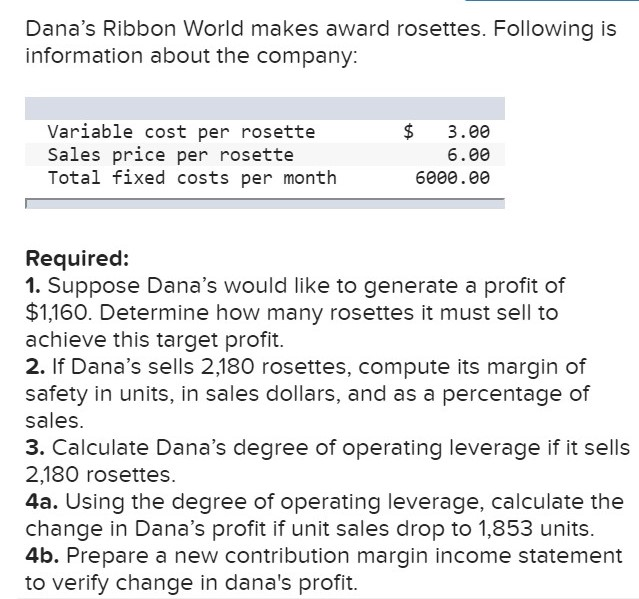# Dana's Ribbon World makes award rosettes. Following is information about the company: Variable cost per rosette Sales price per rosette Total fixed costs per month $3.00 6.00 6000.00 Required: 1. Suppose Dana's would like to generate a profit of$1,160. Determine how many rosettes it must sell to achieve this target profit. 2. If Dana's sells 2,180 rosettes, compute its margin of safety in units, in sales dollars, and as a percentage of sales. 3. Calculate Dana's degree of operating leverage if it sells 2,180 rosettes 4a. Using the degree of operating leverage, calculate the change in Dana's profit if unit sales drop to 1,853 units. 4b. Prepare a new contribution margin income statement to verify change in dana's profit.

Questionhelp_outlineImage TranscriptioncloseDana's Ribbon World makes award rosettes. Following is information about the company: Variable cost per rosette Sales price per rosette Total fixed costs per month $3.00 6.00 6000.00 Required: 1. Suppose Dana's would like to generate a profit of$1,160. Determine how many rosettes it must sell to achieve this target profit. 2. If Dana's sells 2,180 rosettes, compute its margin of safety in units, in sales dollars, and as a percentage of sales. 3. Calculate Dana's degree of operating leverage if it sells 2,180 rosettes 4a. Using the degree of operating leverage, calculate the change in Dana's profit if unit sales drop to 1,853 units. 4b. Prepare a new contribution margin income statement to verify change in dana's profit. fullscreen

### Want to see this answer and more?

Experts are waiting 24/7 to provide step-by-step solutions in as fast as 30 minutes!*